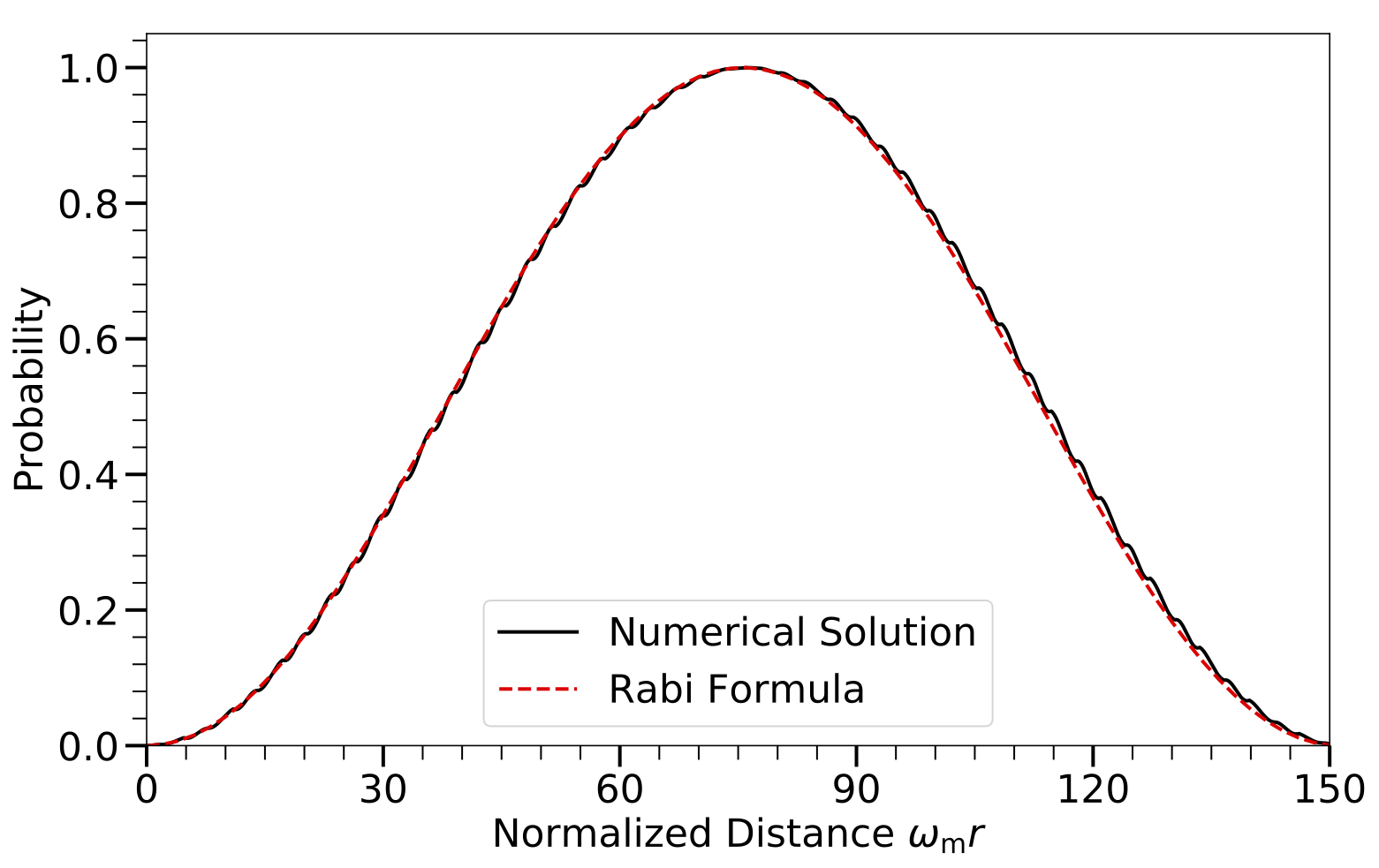# Multi-frequency Matter Profiles Revisited

For a multi-frequency matter profile $$\begin{equation} \lambda(r) = \lambda_0 + \sum_a \lambda_a \cos (k_a r), \end{equation}$$ it is straightforward although a little bit tedious to show that the Hamiltonian in the Rabi basis is $$\begin{equation} \mathsf H^{(\RR)} = - \frac{\omega_\mm}{2} \sigma_3 - \frac{1}{2} \sum_{ \{n_a\} } A_{ \{n_a\} } \begin{pmatrix} 0 & e^{ i K_{\{n_a\}} r }\\ e^{ -i K_{\{n_a\}} r} & 0 \end{pmatrix}, \end{equation}$$ where ${n_a}$ stands for a set of arbitrary integers each associating with a particular Fourier mode, and $$\begin{equation} K_{\{n_a\}} = \sum_{a} n_a k_a \end{equation}$$ and $$\begin{equation} A_{\{n_a\}} = \tan(2\theta_\mm) K_{\{n_a\}} \prod_{a} J_{n_a} \left( \frac{\lambda_{a}}{ k_{a} } \cos(2\theta_\mm) \right). \end{equation}$$ are the wavenumber and amplitude of the Rabi mode denoted by ${n_a}$, respectively. There are potentially many Rabi modes that approximately satisfy the resonance condition $$\begin{equation} K_{\{n_a\}} = \omega_\mm . \end{equation}$$ However, according to the discussion in Sec.~\ref{chap:matter-sec:single-revisted}, the Rabi modes with large $\vert n_a\vert$s have very long oscillation wavelengths and can be ignored even if they are on resonance. The off-resonance Rabi modes with large $\vert n_a\vert$s have very small amplitudes and do not have a significant impact on the resonance either. Therefore, only a finite number of Rabi modes need to be considered for a realistic system.

As an example of a matter profile with multiple frequencies, I will consider the castle wall profile $$\begin{equation} \lambda(r) = \begin{cases} \Lambda_1 &\quad \text{if } -\frac{X_1}{2}+nX\le r\le \frac{X_1}{2}+nX, \\ \Lambda_2 &\quad \text{otherwise}, \end{cases} \label{eq-castle-wall-potential} \end{equation}$$ where $n$ is an arbitrary integer, $X$ is the period of the potential, and $\Lambda_1$, $\Lambda_2$, and $X_1$ are constants. The parametric resonance condition for the castle wall potential has been derived by E. Akhmedov 1: $$\begin{equation} \frac{\tan (\omega_{\mathrm m1}X_1/2)}{\tan (\omega_{\mathrm m2}X_2/2)} = - \frac{\cos 2\theta_{\mathrm m2}}{\cos 2\theta_{\mathrm m1}}, \label{eq-akhmedov-resonance-condition-castle-wall} \end{equation}$$ where $$\begin{equation} \omega_{\mm i} = \omega_\vv \sqrt{ ( \Lambda_i/\omega_\vv - \cos (2\theta_\vv) )^2 + \sin^2 2\theta_\mm }, \end{equation}$$ $$\begin{equation} \theta_{\mm i} = \frac{1}{2} \arctan \left( \frac{\sin (2\theta_\vv)}{ \cos (2\theta_\vv) - \Lambda_i/\omega_\vv} \right) \end{equation}$$ and $X_2 = X_1$. To study the parametric resonance with this particular matter profile, I will decompose the profile into a Fourier series: $$\begin{equation} \lambda(r) = \lambda_0 + \sum_{a=1}^{\infty} \lambda_a \cos\left( k_a r \right), \label{eq-castle-wall-fourier-expanded} \end{equation}$$ where \begin{align*} \lambda_0 &= (\Lambda_1 + \Lambda_2)/2, \\ \lambda_a & = \frac{2}{(2a-1)\pi} (-1)^a \left( \Lambda_1 - \Lambda_2 \right),\\ k_a &= (2a-1)k_1, \\ k_1 &= 2\pi/X, \end{align*} and I have assumed $X_1=X_2 = X/2$. As a concrete example, I choose $\Lambda_1 = 0.3\omega_\vv \cos (2\theta_\vv)$, $\Lambda_2 = 0.7\omega_\vv \cos (2\theta_\vv)$, and $X=2\pi/\omega_\mm=2X_1$. For this particular example, the Rabi mode ${1, 0, 0, \cdots }$ is on resonance. In Fig. Castle Wall Profile, I compared the numerical solution to the full equation of motion and that obtained from the Rabi formula in Eqn.~\eqref{app:rabi-system-transition-probability}. They agree with each other very well.Castle Wall ProfileThe transition probability of the neutrino flavor conversion with a castle wall matter profile as a function of distance $r$. The period of the castle wall potential $X$ is chosen that the Rabi mode with wavenumber $K=1$ is on resonance.

In the above example, there are an infinite number of Rabi modes that are on resonance in addition to ${1,0,0, \cdots}$. In principle, one should add the amplitudes of all these resonances modes together in calculating the transition probability with the Rabi formula. However, all the Rabi modes that are on resonance have very tiny amplitudes except ${1,0,0,\cdots}$ (see Table castle-wall-relative-detunings-some). Therefore, they can be neglected in calculations. There are also an infinite number of Rabi modes that are off resonance. In Table castle-wall-relative-detunings-some I listed some of these Rabi modes with the largest amplitudes. One can see that the change to the relative detunings $\RD_{({n_a})}$ of the on-resonance mode ${1,0,0,\cdots}$ due to the presence of the mode ${n_a}$ are all very small. As a result, they all have very little impact on the neutrino flavor conversion. This explains the agreement between the numerical solution and the result using the Rabi formula in Fig. Castle Wall Profile.

${n_a}$$A_{{n_a}}/A_{{1,0,0,\cdots}}$$\RD_{{n_a}}$$\Delta \RD_{({n_a})} {-2, 1, 0,\cdots}$$5.4\times 10^{-4}$0-
${-1, -1, 1,\cdots}$$4.3\times 10^{-5}0- {0, 2, -1,\cdots}$$2.4\times 10^{-6}$0-
${-1,0, 0, \cdots}$$1$$48$$1.0\times 10^{-2} {0,1, 0, \cdots}$$0.33$$1.5\times 10^2$$1.1\times 10^{-3}$
${2,0, 0, \cdots}$$1.3\times 10^{-3}$$2.4\times 10^{2}$$2.0\times 10^{-4}$

Table castle-wall-relative-detunings-some shows the amplitudes and relative detunings of a few Rabi modes ${n_a}$ and the changes to the relative detunings of the on-resonance modes due to these Rabi modes if they are off resonance.

1. E. Kh Akhmedov, "Parametric resonance in neutrino oscillations in matter", Pramana 54, 47-63 (2000) . ↩︎